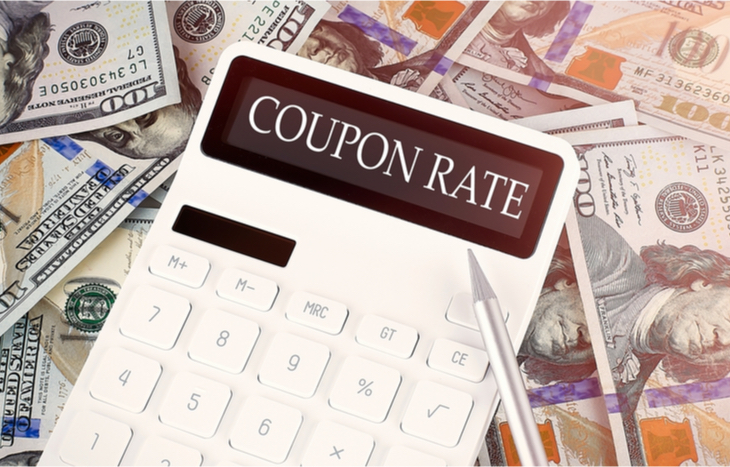Home Investing What is a Coupon Rate?

# What is a Coupon Rate?

When considering bonds, issuers and investors alike need to consider the coupon rate: the interest paid by the bond. Most bonds pay out coupons on a semi-annual basis, relative to the par (face) value of the bond. It’s a representation of how much the issuer will pay to borrow money, as well as how valuable the bond will be to investors over its life.

The bond’s coupon rate is especially important when considering bonds as fixed-income investments. The higher the rate, the more the bond will pay out each year. Investors in possession of high-interest rate bonds will see higher semi-annual payments. This can raise the value of their bond based on the current yield curve.

Coupon rate is a major variable for bond investors to consider. Here’s what you need to know and how the rate affects the entire trajectory of a fixed-income investment.## How to Calculate Coupon Rate

Calculating a bond’s coupon rate comes down to examining its par value and its yield. Specifically, investors would divide the sum of annual interest payments by the par value:

Coupon Rate = Total Coupon Payments / Par Value

For example, if a company issues a \$1,000 bond with two \$25 semi-annual payments, its coupon rate would be \$50/\$1000 = 5%. Rates come fixed from the point of issuance; still, it’s important to understand how to calculate them when evaluating fixed-income investments through different lenses, such as Yield to Maturity (YTM).

## How Coupon Rate Affects Demand

Coupon rates play a significant role in dictating demand for certain bonds. They come fixed at the time of issuance, while interest rates change. This means the two work in tandem to drive bond prices—and thus, demand for bonds.

• If the rate is higher than the current interest rate, bonds will trade at a premium.
• If the rate is lower than the current interest rate, bonds will trade at a discount.

Investor demand for bonds is higher when the guaranteed interest payment on a bond is higher than the payout on newly issued bonds. The face value for a \$1,000 high-coupon bond may trade at \$1,100 if its 3% coupon is higher than the current 2.5% interest rates. Likewise, that same bond might trade for \$900 if the current prevailing interest rate is 4.2%.

Coupon rates are the static variable in a dynamic bond market. This makes them an important variable in establishing market rates.

## The Inverse Relationship Between Price and Yield

As bond prices fluctuate and coupon rates stay the same, the yield of a bond changes. This is an extremely important consideration because it changes the value of a bond.

• At face value, a bond’s yield equals its coupon rate.
• At a premium, a bond’s yield is lower than its coupon.
• And at a discount, a bond’s yield is higher than its coupon.

For example, a \$1,000 bond with a 4% coupon will pay \$40 per year (two \$20 payments)—a yield of 4%. However, if that bond trades at \$1,100 with the same coupon, its yield drops: \$40/\$1,100 = 3.63%. Conversely, if that bond trades at \$900 with the same coupon, its yield rises: \$40/\$900 = 4.44%.

As the price of a bond rises and falls, so does its yield: inversely. This is the mechanism behind fluctuations in demand.

## Effective Yield vs. Nominal Yield

The most important part of a fixed-income investment is its ability to generate returns in the form of coupon payments. To measure this ROI, investors can look at both effective yield and nominal yield.

• Effective yield is the ROI of a bond with interest payments reinvested at the same rate.
• Nominal yield is the ROI of a bond based on its coupon rate, with no reinvestment.

Most investors look to the effective yield as the more accurate representation of a bond’s yield. However, those investors who rely on coupon payments as a form of passive income would look to nominal yield as a better measure, since coupon payments aren’t reinvested. Another, more comprehensive way of looking at this, is to consider coupon rate vs. yield to maturity.

## Coupon Rate vs. Yield to Maturity

One of the most important metrics to use a bond’s coupon rate is Yield to Maturity (YTM). Because the coupon remains fixed, investors can use it to calculate the total yield of a bond if held to maturity, assuming all interest payments.

For long-term investors, coupon rate is a more important factor than YTM. This is because they’re more likely to depend on the interest payouts of the bond. Therefore, a higher coupon means a higher payment. Conversely, bond traders prefer YTM because they’re acquiring bonds in a secondary market, where carrying value matters more.

In either case, it’s smart for bond investors to consider both the rate and the YTM. Both metrics offer a look at bond value in the context of different investment theses.

## Coupon Rates Dictate Bond Value

Investors focused on fixed-income securities need to put special emphasis on coupon rate. Not only is it an important factor in the current trading price of a bond, it also affects more important measures, such as YTM. It’s the constant variable among many others that are ever-changing.

Coupon payments are a source of profit, which makes the coupon of special importance to investors. Whether you’re purchasing a bond that’s hot off the presses or trading in a secondary market to acquire bonds at a premium or discount, coupon rate is the prevailing factor to consider.